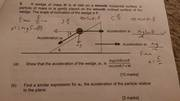Applied Mathematics Problem

Member warned that the homework template must be used
Hi! New to this forum and signed up because I've just started a foundation degree in mechanical engineering, and having been out of education for a very long time beforehand, getting back to grips with mathematics and the like!

Anyway, I would like to ask for some advice on this problem, I know how the top part of the fraction come about, as well as the lower case and upper case m in the lower part of the fraction, but still unsure as to why sin(theta) is squared? Thanks in advance!Attachments

Last edited by a moderator:

We need to work the whole solution out to tell where does that ##\sin^2 \theta## came from.
So a better way would be that you let us, how you worked out the question and we try to solve your confusion.

Hi and thanks for your reply, I have got as far as identifying that the equation shown for a1 is a rearranged F=ma, to a=F/m , the F being mgsin0cos0 and the m being m the lower part of the fraction.

I know m is the mass of the particle on the wedge and M is the mass of the wedge but fail to see how sin0^2 fits in, I would imagine it would be to so with calculating the mass.

Hi and thanks for your reply, I have got as far as identifying that the equation shown for a1 is a rearranged F=ma, to a=F/m , the F being mgsin0cos0 and the m being m the lower part of the fraction.

I know m is the mass of the particle on the wedge and M is the mass of the wedge but fail to see how sin0^2 fits in, I would imagine it would be to so with calculating the mass.
Did you draw a FBD ?

Here is what I drew, and how far I got. As I understand the surfaces are frictionless so the round particle will fall straight down and the wedge will move to the right.
Normal reaction of sphere on the block is not ##mg\cos\theta##, It is so because the big block is accelerating.

Equate the forces perpendicular to the incline acting on the sphere, from there you will get the correct value of normal reaction. Substitute it in your last equation and you will get the answer.

haruspex